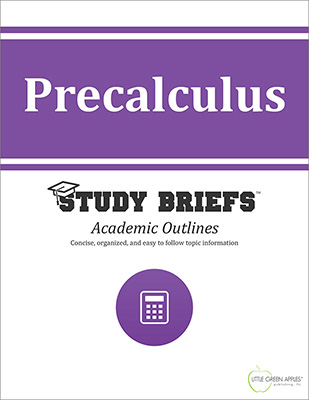# PrecalculusLook inside
• Format: eBook
• Edition: 2016
• Publisher: Little Green Apples Publishing LLC

Price: \$4.99

## Description:

The Precalculus Study Brief contains a useful review of foundational mathematical concepts and techniques that are needed to prepare for learning Calculus. This academic outline offers coherent definitions, straightforward explanations, and numerous instructive examples that are easy to follow and simple to understand. This Study Brief is intended as a quick reference to prepare students who are ready to advance into higher-levels of high school orr college mathematics. It is also a convenient refresher for adult learners interested in a condensed summary of the fundamentals required to comprehend and to be able to solve basic calculus problems. Sample equations and formulas are included in an organized and highly visual format. Example problems with worked-out answers serve as at-a-glance reference information for future independent problem solving. This Study Brief emphasizes basic functions that are needed before moving on to use more advanced calculus methods. Individual sections cover linear functions, polynomial functions, quadratic functions, rational functions, exponential functions, logarithmic functions, trigonometric functions and periodic functions. Explanation of sequence and series, including properties of summation notation is also included. Applications of trigonometry and analytic geometry are covered.

## Topics Covered:

• Functions
• Linear Functions
• Polynomial Functions